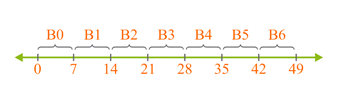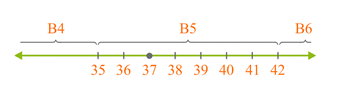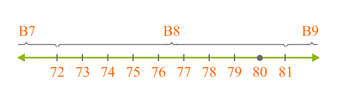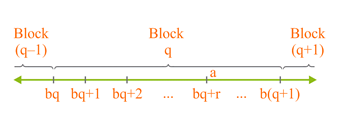# Euclid's Division Lemma

Go back to  'Arithmetic-Integers'

## Introduction to Division of Integers:

Let's begin with a famous problem/riddle:

A trader was moving along a road selling eggs. An idler who didn’t have much work to do started to get the trader into a wordy duel. This grew into a fight, he pulled the basket with eggs and dashed it on the floor. The eggs broke. The trader requested the Panchayat to ask the idler to pay for the broken eggs. The Panchayat asked the trader how many eggs were broken. He gave the following response:

• If counted in pairs, one will remain;

• If counted in threes, two will remain;

• If counted in fours, three will remain;

• If counted in fives, four will remain;

• If counted in sixes, five will remain;

• If counted in sevens, nothing will remain;

• My basket cannot accommodate more than 150 eggs.

The problem, of course, is to find the number of eggs in the basket. This is a very good example of the concept of division of two integers, to obtain a quotient and a remainder. Let us solve this problem step by step.

Let the number of eggs be $$x$$. Note that $$x$$ must be a multiple of 7, that is:

$x = 7a,\;a \in \mathbb{Z}$

Note that if counted in pairs, one egg remains, that is:

$x = 2b + 1,\;b \in \mathbb{Z}$

In other words, $$x$$ is odd. Let us write out all odd multiples of 7 which are less than 150:

7,  21,  35,  49,  63,  77,

91,  105,  119,  133,  147

Also, note that $$x$$ is not a multiple of 3 or 5. Thus, we cross out all multiples of 3 or 5 on our list:

7,  21,  35,  49,  63,  77,

91,  105,  119,  133,  147

We now have 6 possible numbers on our list $$\left( {7,49,77,91,119,133} \right)$$. If counted in fours, three will remain, which means that $$x$$ must be one less than a multiple of 4. Thus, we cross out all those numbers which are not one less than a multiple of 4:

7,  4977,  91,  119,  133

Left possible numbers are $$\left( {7,91,119} \right)$$.

Also, $$x$$ should be one less than a multiple of 3, and one less than a multiple of 5, and so:

791,  119

Therefore, the number of eggs in the basket are $$\boxed{119}$$.

You can verify that the number 119 satisfies all the conditions of the problem:

$119 = \left\{ \begin{array}{i}2\left( {59} \right) + 1\\3\left( {39} \right) + 2\\4\left( {29} \right) + 3\\5\left( {23} \right) + 4\\6\left( {19} \right) + 5\\7\left( {17} \right) + 0\end{array} \right.$

What we have seen here is an example of division of integers!

✍Note: When you divide one integer by another non-zero integer, you are left with a quotient and a remainder. For example, when 119 is divided by 4, the quotient is 29 and the remainder is 3.

Let us formalize this discussion by Euclid's Division Lemma.

## What is Euclid's Division Lemma?

Euclid’s Division Lemma (lemma is like a theorem) says that given two positive integers $$a$$ and $$b$$, there exist unique integers $$q$$ and $$r$$ such that:

$\boxed{a = bq + r,\;0 \leqslant r < b}$

The integer $$q$$ is the quotient, and the integer $$r$$ is the remainder. The quotient and remainder are unique.

In simple words, Euclid's Division Lemma says that whenever you divide an integer by another (non-zero) integer, you will get a unique quotient integer and a unique remainder integer.

Here are some examples of the application of this lemma:

\begin{align}&\underline {17} = \underline 3 \left( 5 \right) + 2\\&\underline {24} = \underline 7 \left( 3 \right) + 3\\&\underline {61} = \underline {11} \left( 5 \right) + 6\\&\;\;\underline 7 = \underline {12} \left( 0 \right) + 7\end{align}

In each line, the underlined numbers are the dividend and divisor respectively, the number in brackets is the quotient, while the last number is the remainder.

✍Note: The remainder is always less than the divisor.

## A visual model for Euclid's Division Lemma

Consider an arbitrary integer, say 37. Suppose that you divide this by 7. What will be the quotient and the remainder? We have:

$37 = 7\left( 5 \right) + 2$

Clearly, the quotient is 5 and the remainder is 2.

Let us interpret this physically. Let us divide the number line into blocks of 7 units as shown below:We have named each block as shown in the figure. In which block will 37 lie? It will lie in B5, which means that between this block and the number 0, there are 5 intervening blocks (B0 to B4). This corresponds to the quotient.

What is the interpretation of the remainder? For that, zoom-in block B5, which will be 7 units long:Cleary, 37 lies 2 units from the start of its block (which is B5). This corresponds to the remainder.

Let us repeat this process again. Suppose that we have to divide 80 by 9. This time, we divide the number line into blocks of 9 units each. The number 80 will lie in block B8, 8 units from the start of its block:Thus, the quotient, in this case, is 8, and the remainder is 8.

Finally, let us generalize this. Let $$a$$ and $$b$$ be any positive integers. Suppose that we divided $$a$$ by $$b$$. We will obtain a quotient $$q$$ and a remainder $$r$$. We can write this fact as follows:

$\boxed{a = bq + r,\;0 \leqslant r < b}$

Physical interpretation: we divide the number line into blocks of $$b$$ units each. Then, the number a will lie in block number q, at a distance of r units from the start of the block:This is, as we have seen earlier, Euclid’s Division Lemma: Given positive integers $$a$$ and $$b$$, there exist unique integers $$q$$ and $$r$$ such that:

$a = bq + r,\;0 \le r < b$

That the quotient and remainder are unique should be obvious. Also, the remainder must be less than the divisor. Can you see why? Refer to the last figure above. Note how each block is of length $$b$$ units, and how the remainder can only take values from a minimum of 0 (start of the block) to a maximum of $$b - 1$$ (one unit less than the end of the block).Challenge 1: Does Euclid’s Division Lemma hold if $$a$$ is a negative integer?

⚡Tip: Actually try by taking a negative integer as $$a$$ and any integer as $$b$$.

## Solved Examples:

Example 1. An odd positive integer $$n$$ is written as follows:

$n = 4k + m$

What values can $$m$$ take?

Solution. If you divide $$n$$ by 4, we will have (by Euclid’s Division Lemma):

$n = 4k + m,\;m = 0,\;1,\;2,\;3$

Thus, $$m$$ can take four values: 0, 1, 2, or 3. However, it should be clear that if $$m$$ is 0 or 2, then $$n$$ will be even, whereas we want $$n$$ to be odd. This means that $$m$$ can take only two values: 1 and 3.

In other words, any odd positive integer $$n$$ can be written as

$\boxed{n = 4k + 1}\;\;\;\;\;{\text{or}}{\mkern 1mu} {\mkern 1mu} {\mkern 1mu} {\mkern 1mu} {\mkern 1mu} {\mkern 1mu} {\mkern 1mu} {\mkern 1mu} {\mkern 1mu} {\mkern 1mu} {\mkern 1mu} \boxed{n = 4k + 3}$Challenge 2: Show that any positive even integer is of the form $$6q$$, $$6q + 2$$, or $$6q + 4$$, where $$q$$ is some integer.

⚡Tip: Use Euclid’s Division Lemma with a similar approach as in example 1.

Example 2.  Let $$n$$ be an odd integer. Show that $${n^2} - 1$$ is a multiple of 8.

Solution. By Euclid’s Division Lemma, $$n$$ can be written as

$n = 2k + 1,\;k \in \mathbb{Z}$

Thus,

\begin{align}&{n^2} - 1 \;= {\left( {2k + 1} \right)^2} - 1\\& \qquad\quad= \left( {4{k^2} + 4k + 1} \right) - 1\\& \qquad\quad= 4{k^2} + 4k\\&\qquad\quad = 4k\left( {k + 1} \right) & \end{align}

Note that the product $$k\left( {k + 1} \right)$$ will always be even. This is because either $$k$$ is even, or if not, then $$k + 1$$ is even.

Thus,

$\boxed{n{\text{ must be a multiple of }}8}$

Example 3. The cube of a positive integer is divided by 9. What can be the possible remainders?

Solution. This is an interesting problem. Somehow, we have to use Euclid's Division Lemma, and we have to figure out how. If we can express the cube of any positive integer in the form $$9q + r$$, then our problem simplifies: we then have to find what values $$r$$ can take.

Let $$n$$ be any positive integer. Note that on division of $$n$$ by 3, we can get three possible remainders: 0, 1 or 2. Thus, $$n$$ can be written as:

\begin{align}&n = 3k\\&\quad{\rm{or}}\\&n = 3k + 1\\&\quad{\rm{or}}\\&n = 3k + 2\end{align}

We consider each case separately.

Case 1: In this case, we have:

${n^3} = {\left( {3k} \right)^3} = 27{k^3} = 9\left( {3{k^3}} \right)$

Thus, the cube of $$n$$ will be perfectly divisible by 9 in this case (the remainder will be 0).

Case 2: In this case, we have:

\begin{align}&{n^3} = {\left( {3k + 1} \right)^3}\\&\quad = {\left( {3k} \right)^3} + {\left( 1 \right)^3} + 3\left( {3k} \right)\left( 1 \right)\left( {3k + 1} \right)\\&\quad= 27{k^3} + 1 + 9k\left( {3k + 1} \right)\\&\quad = 9k\left( {3{k^2} + 3k + 1} \right) + 1\\&\quad = 9\left\{ {k\left( {3{k^2} + 3k + 1} \right)} \right\} + 1 & \end{align}

Clearly, on dividing the cube of $$n$$ by 9 in this case, we will be left with a remainder of 1.

Case 3: In this case, we have:

\begin{align}&{n^3} = {\left( {3k + 2} \right)^3}\\&\quad= {\left( {3k} \right)^3} + {\left( 2 \right)^3} + 3\left( {3k} \right)\left( 2 \right)\left( {3k + 2} \right)\\&\quad = 27{k^3} + 8 + 18k\left( {k + 2} \right)\\&\quad= 9k\left( {3{k^2} + 2k + 4} \right) + 8\\&\quad = 9\left\{ {k\left( {3{k^2} + 2k + 4} \right)} \right\} + 8 & \end{align}

On dividing the cube of $$n$$ by 9 in this case, we will evidently be left with a remainder of 8.

Thus, we conclude that dividing the cube of a positive integer by 9 can yield three possible remainders:

$\boxed{{\text{Three Possible Remainders: }}0,1,8}$

In other words, the cube of any integer can be expressed as follows:

\begin{align}&{n^3} = 9k\\&\quad\;{\rm{or}}\\&{n^3} = 9k + 1\\&\quad\;{\rm{or}}\\&{n^3} = 9k + 8\end{align}

You may verify this result by taking some cubes and checking the remainders you obtain upon their division by 9.Challenge 3: The cube of a positive integer is divided by 3. What can be the possible remainders?

⚡Tip:  Let $$n$$ be any positive integer then it is of the form $$3k$$, $$3k + 1$$ or $$3k + 2$$. Now square each of these.

Arithmetic Integers
grade 10 | Questions Set 2
Arithmetic Integers
Arithmetic Integers
grade 10 | Questions Set 1
Arithmetic Integers